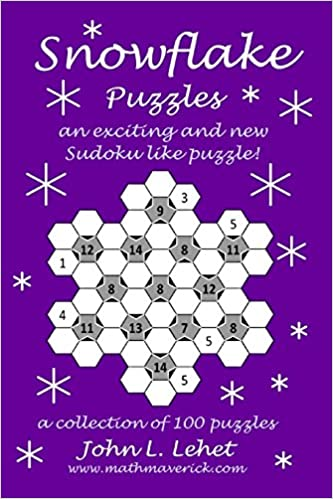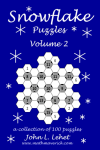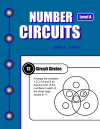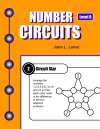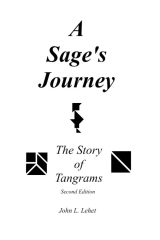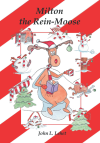Day 25 - MasterMind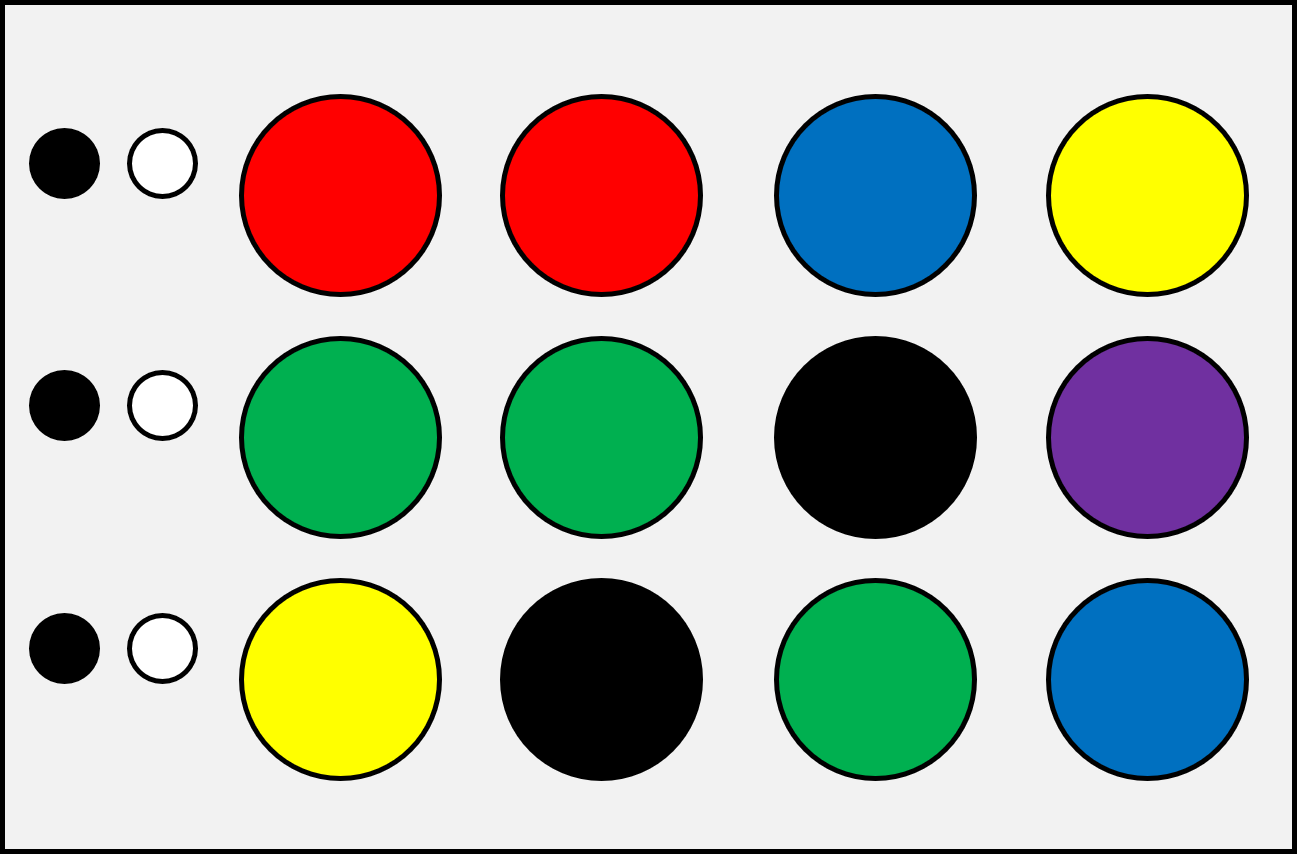Day 2 - Letter Riddles
Letter Riddles are simply stated riddles with solutions that are letters. For example:

What two letters are a girl's name?

There are multiple answers, like KT as in Katie, ME as in Emmie or GG as in Gigi. When you say the letters you get the name!

Here are five for you to try - Good Luck!

 What two letters are sometimes poison? What two letters are jealous? What two letters are too much? What two letters are not hard? What two letters are some?
Day 3 - Missing Word Puzzles
In each puzzle, a word or words are missing, replaced by their first letter. To solve each puzzle, you need to find the missing words. Here’s an example of how they work:

example: 12 I in a F     has solution     12 INCHES in a FOOT

 1 W on a U 2 N in a D 3 F in a Y 4 S in a D of C 5 O in the W 6 F on a C SW and the 7 D 8 L on an O 9 L of a C 10 Y in a D
Day 4 - SET
In the game SET, each card has four attributes - color, shape, number and fill. A set is comprised of 3 cards in which each attribute is all the same or all different across all 3 cards.

The rule is "IF two are the same is one is different, THEN it's NOT a SET!"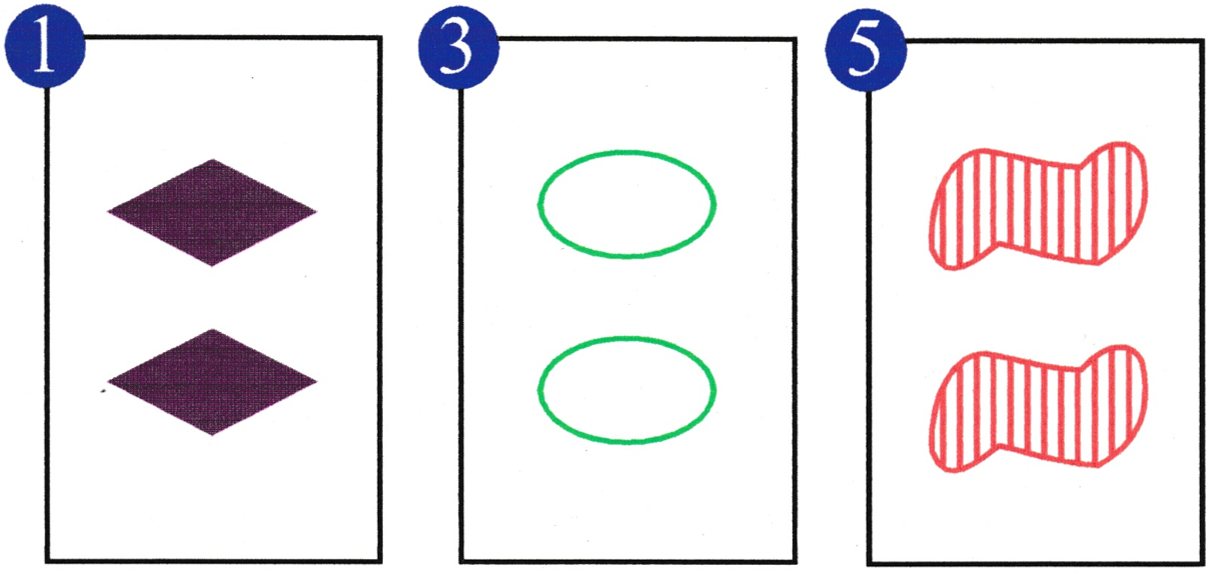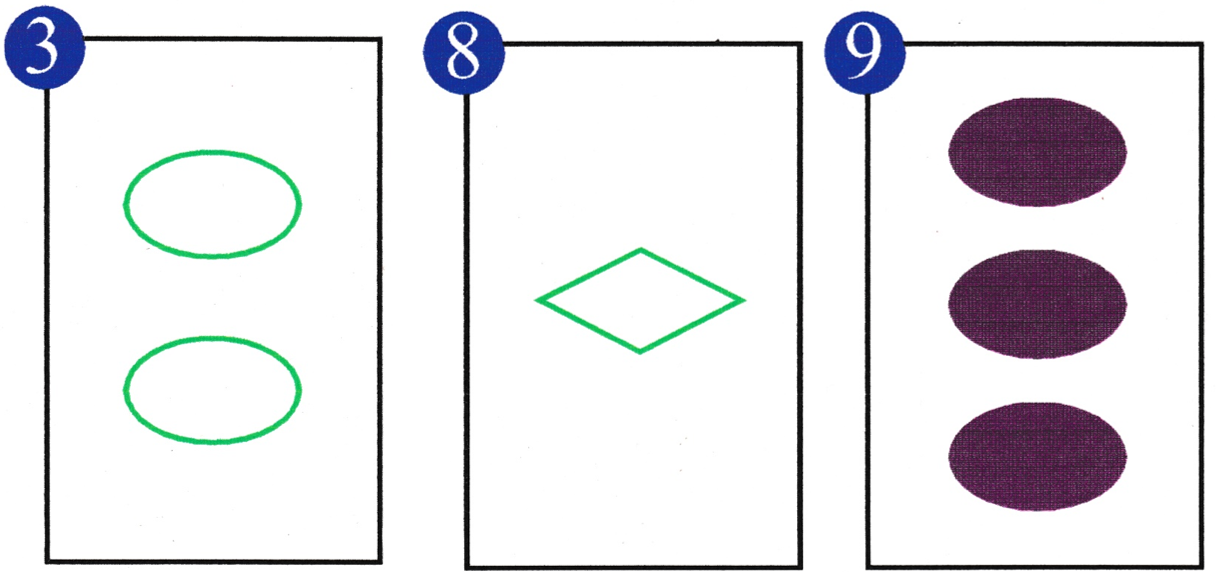This is a setthe colors are all differentthe shapes are all differentthe numbers are all the samethe fills are all different This is NOT a setthe numbers are all differentbut there are ... two greens and one purpletwo ovals and one diamondone solid and two empty fills

Can you find 12 sets in the following nine cards? Click on a card to select it! You will be automatically scored and order does not matter!

 1 2 3 4 5 6 7 8 9 10 11 12
Day 5 - Rebus Puzzles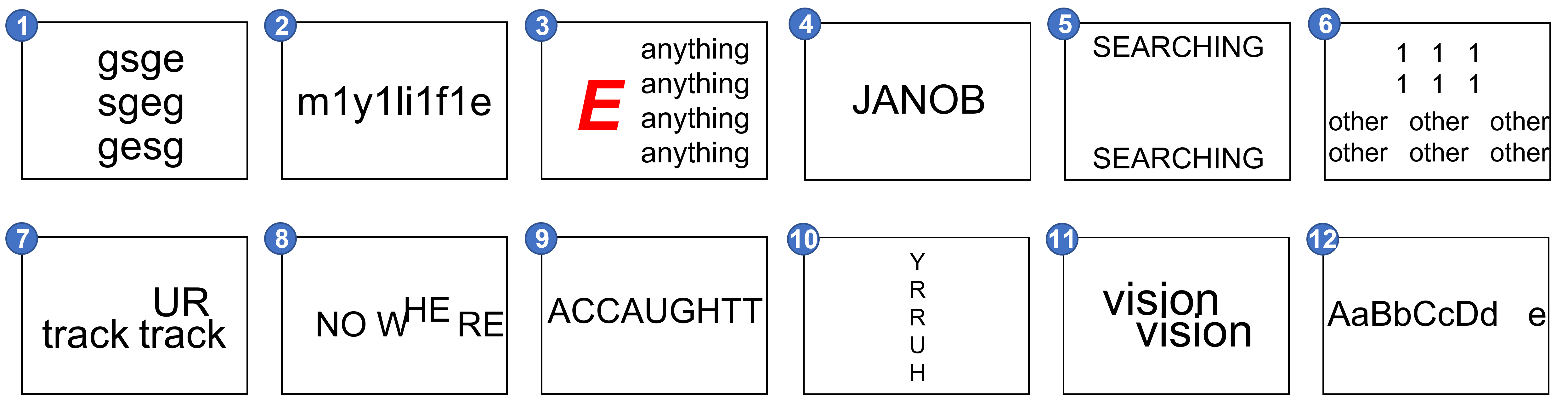1 2 3 4 5 6 7 8 9 10 11 12
Day 6 - Alphametics
Alphametics are puzzles that use simple arimetic in which letters represent unique single digit numbers. The trick is to determine the number to which each letter corresponds. Below there are eight distinct letters, O, N, E, T, W, F, U and R. Use the numbers 0 through 9 (two of which will NOT be used) to solve the puzzle.Day 7 - Counting Squares
Today’s puzzle is a counting puzzle. The objective is to count the number of squares in each figure. After completing all three puzzles, select Check to see if you're right! Although this looks simple, this may prove to be more challenging than it looks.

As a hint, the number of squares in the Puzzle 1 is NOT 4!
Good Luck!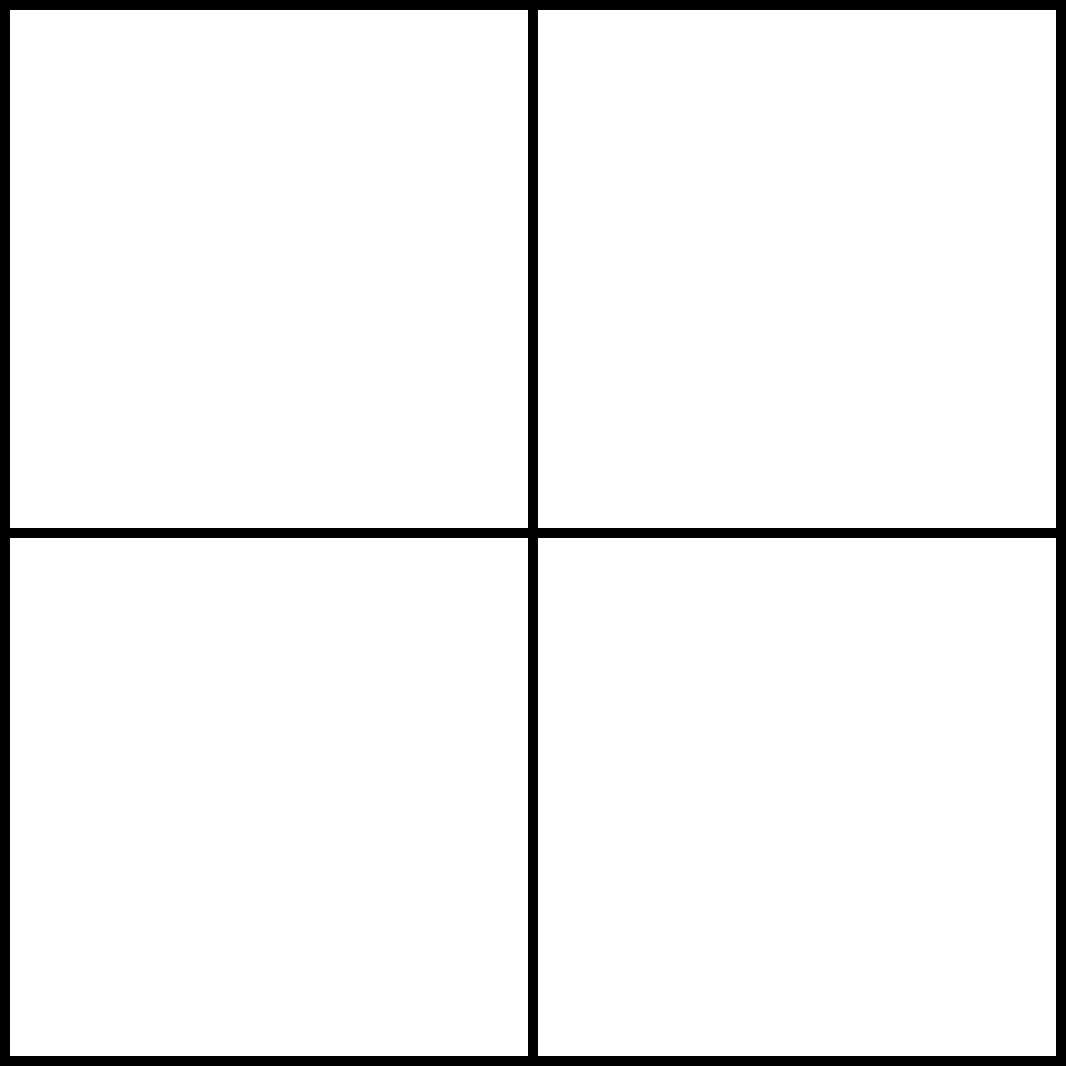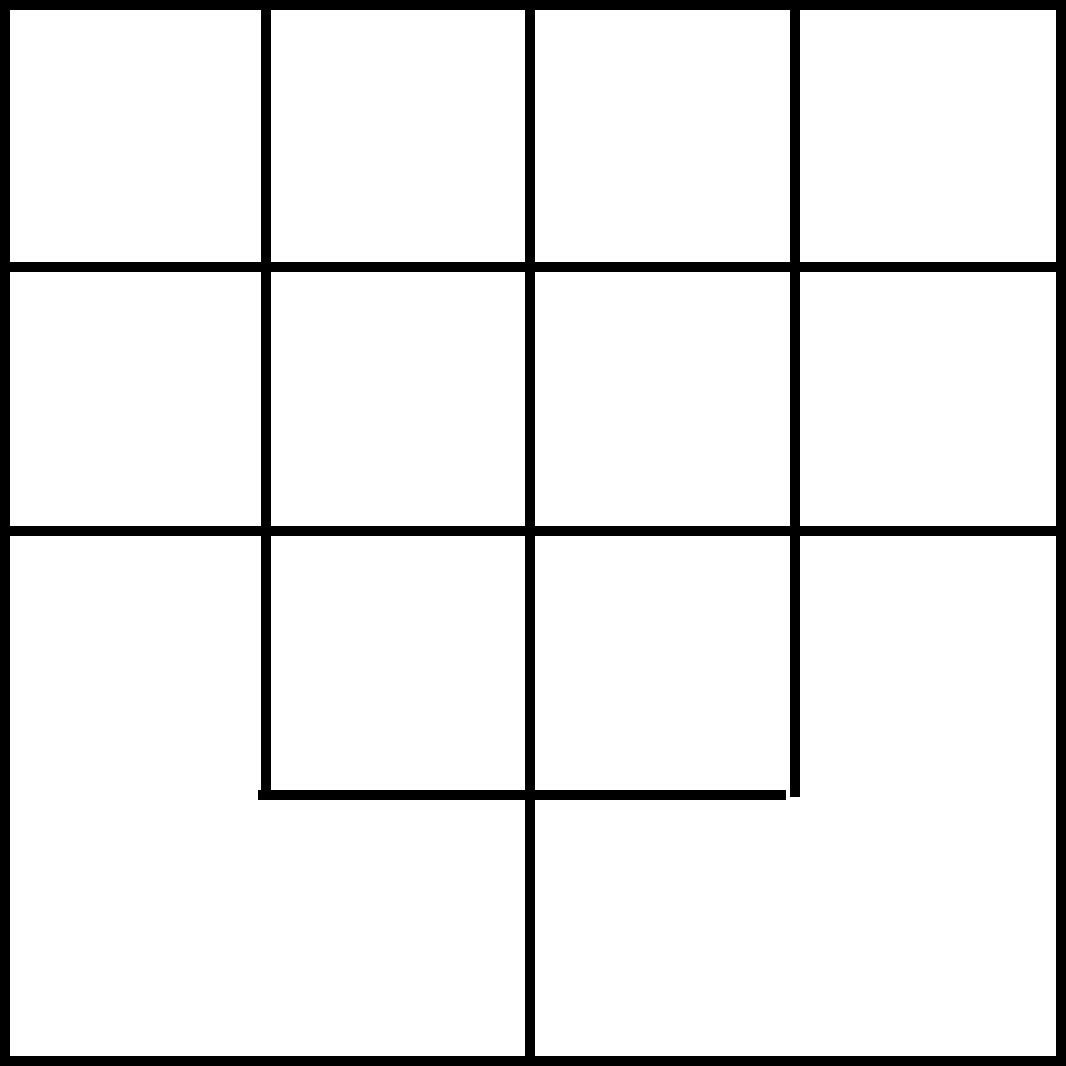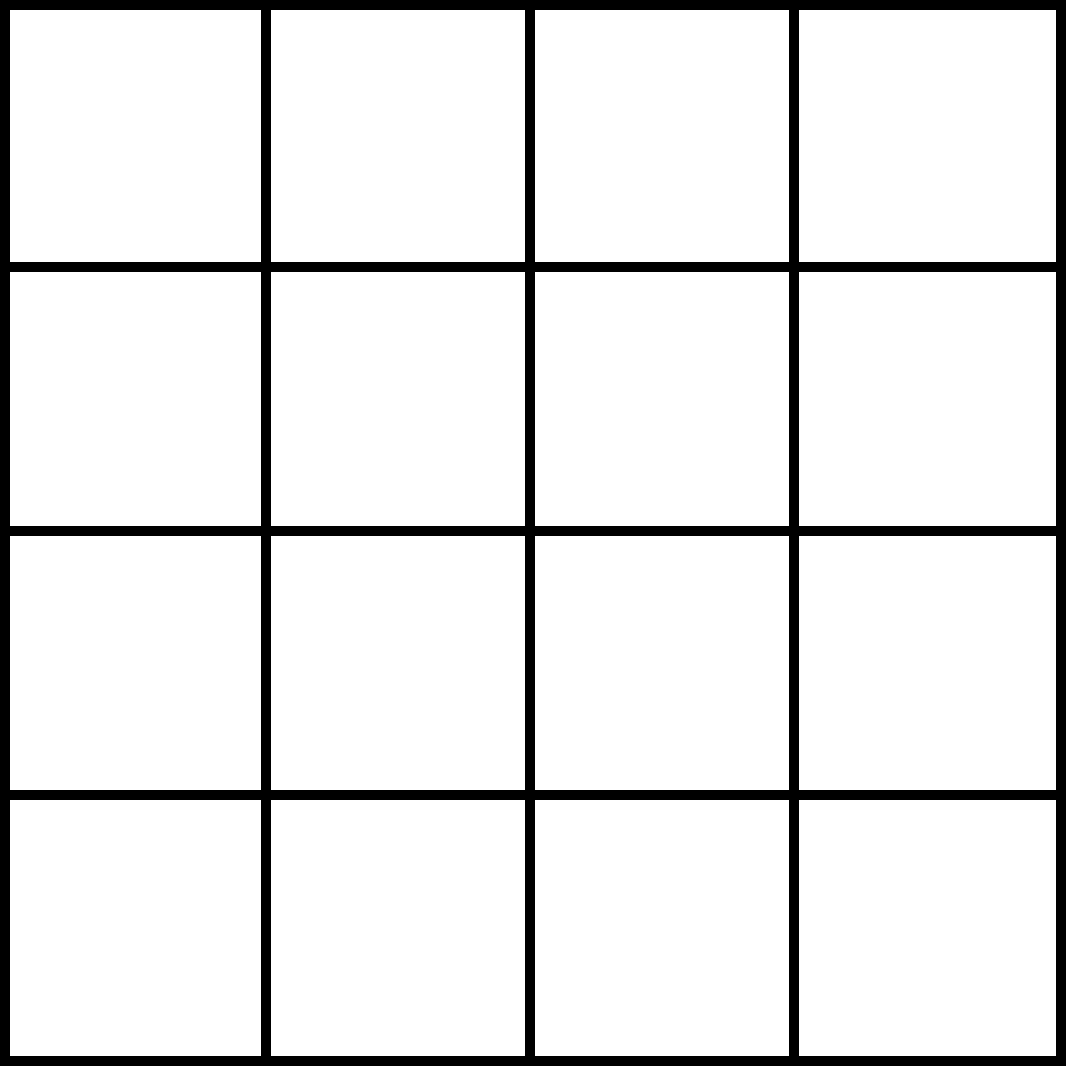Day 1 - Even's Only
Use each of the numbers 2, 4, 6 and 8 once and only once along with any combination of the arithmetic operators + - * / and at most one set of parenthesis ( ) to make each number 1 through 10.

For example, here are two ways to make the number 10

8 * 4 / 2 - 6 and (8 - 6) * 4 + 2

Just enter your solutions in the boxes below and select Check! Good Luck!

 1 = 2 = 3 = 4 = 5 = 6 = 7 = 8 = 9 = 10 =
Day 8 - Odd's Only
Use each of the numbers 3, 5, 7 and 9 once and only once along with any combination of the arithmetic operators + - * / and at most one set of parenthesis ( ) to make each number 1 through 10.

For example, here are two ways to make the number 8

9 - 3 - 5 + 7 and 5 * 7 - 3 * 9

Just enter your solutions in the boxes below and select Check! Good Luck!

 1 = 2 = 3 = 4 = 5 = 6 = 7 = 8 = 9 = 10 =
Day 9 - Number Sense - Primes
use the numbers 0 1 2 3 4 5 6 7 8 9, once and only once,
to fill in the eight boxes below
answers may be one-digit or two-digit numbers

 an evennumber a powerof 2 a powerof 3 a primenumber a prime withprime digitswhose sumis prime a powerof 5 a powerof 7 the product oftwo primes

primes = {2,3,5,7,11,13,17,19,23,29,31,37,41,43,47,53,59,61,67,71,73,79,83,89,97}
powers of 2 = {1,2,4,8,16,32,64}
powers of 3 = {1,3,9,27,81}
powers of 5 = {1,5,25}
powers of 7 = {1,7,49}
0 is an even number!
Day 10 - More Missing Word Puzzles
Just like in Day 3, in each puzzle a word or words are missing, replaced by their first letter. To solve each puzzle, you need to find the missing words. Here’s an example of how they work:

example: 12 I in a F     has solution     12 INCHES in a FOOT

 2 P in a P 2 H in a W 3 A in a T 4 ES in a S 5 F on each H 7 D in a W 10 D in a D 12 M in a Y 60 M in an H A the W in 80 D
Day 11 - What Goes Where?
Using the numbers 1,2,3,4,5,6,7,8 and 9 once and only once,
use the clues below to solve the puzzle.

 1. the center row contains only even numbers 2. the bottom row contains only odd numbers 3. 4 is in the top row 4. the top row contains the smallest number in each column 5. 2 is in the center circle 6. the center column contains the smallest number in each row 7. the left column contains only multiples of 3

Day 12 - Self-Referential Puzzle
Answer each of the following questions so that all are correct!

 1. How many questions have an odd solution? 1 2 3 4 2. How many questions have an even solution? 1 2 3 4 3. How many questions have 1 as the solution? 1 2 3 4 4. How many questions have 2 as the solution? 1 2 3 4 5. How many questions have 3 as the solution? 1 2 3 4 6. How many questions have 4 as the solution? 1 2 3 4
Day 13 - Math Riddles
Answer each with number or a single word!

Good Luck!

 What 4-digit number becomes a palandrome when 1 is either added OR subtracted? What is the only number whose name is the number of letters in it? What is the only number whose name is twice the number of letters in it? Cats have four, you have two and this has three! What can this be? If a foot and a year were the same, then an inch could go by this name?
Day 14 - NOB Puzzle
In the puzzle below, the six right-most numbers in the bottom row are a combination of two numbers, the number to the left and the number above. This is illustrated with the two arrows that point to these numbers.

For example, 24 (the second number in the bottom row) is a combination of 64 (above) and 40 (to the left). Similarly, 44 is a combination of 68 and 24. Given these two examples, the combination looks like subtraction, 64-40 = 24 and 68-24 = 44. But it's NOT simple subtraction!

To solve the puzzle, all you need to do is fill in the empty red square in the bottom row. Be assured that the solution is NOT 35! Just combine two numbers (using simple arrithmetic) to find the third number!
Good Luck!

 64 68 16 63 46 31 ↓ ↓ ↓ ↓ ↓ ↓ 40 → 24 → 44 → 28 → → 48 → 20
Day 15 - Primes Only
Use each of the numbers 2, 3, 5 and 7 once and only once along with any combination of the arithmetic operators + - * / and at most one set of parenthesis ( ) to make each number 1 through 10.

For example, here are two ways to make the number 10

(3 + 7) / 2 + 5 and 3 * 5 - 7 + 2

Just enter your solutions in the boxes below and select Check! Good Luck!

 1 = 2 = 3 = 4 = 5 = 6 = 7 = 8 = 9 = 10 =
Day 16 - Number Sense - Factors
use the numbers 0 1 2 3 4 5 6 7 8 9, once and only once,
to fill in the eight boxes below
answers may be one-digit or two-digit numbers

 a factorof 15 a factorof 42 a factorof 20 a factorof 28 a factorof 29 a factorof 8 a factorof 25 a factorof 44
Day 17 - Missing Word Puzzles
Just like in Days 3 and 10, in each puzzle a word or words are missing, replaced by their first letter. To solve each puzzle, you need to find the missing words. Here’s an example of how they work:

example: 12 I in a F     has solution     12 INCHES in a FOOT

 3 P for a FG 3 WK 3 LP and the BBW 3 S (M, L and C) 3 H on a T GL and the 3 B 3 W on a T 3 is a C 3 BM 3 FH
Day 18 - Problems for the Ages
1. Mr Dills had three daughters, all born on the same date, but in consecutive years.
At their birthday party this year, Mr Dills noted that the girls were 3, 4 and 5 years old respectively.

How old will the girls be the next time their ages will be multiples of 3, 4 and 5 respectively?

 youngest oldest

2. Two brothers were born on the same day, exactly two years apart. In 1979, at their birthday party, the older brother commented, "Wow, this is something. The product of our current ages equals the year between the years in which we were born!"

How old are the brothers?

 younger older

Day 19 - Another Alphametic Puzzle
Alphametics are puzzles that use simple arimetic in which letters represent unique single digit numbers. The trick is to determine the number to which each letter corresponds. Below there are eight distinct letters, F, O, U, R, N, E, I and V. Use the numbers 0 through 7 (DO NOT use 8 and 9) to solve the puzzle.Day 20 - Heteronyms
Heteronyms are words with the same spelling, but different meaning and pronunciation.
A great examaple is putting

1. he is putting the can on the shelf.
2. he is putting the ball on the golf green.

Below are five puzzles all with a one word heteronym solution! Good Luck!

 eye discharge rip tiny hour fraction type of fish musical instrument shut near gift accessory respectfully bend forward
Day 21 - Another What Goes Where?
Using the numbers 1,2,3,4,5,6,7,8 and 9 once and only once,
use the clues below to solve the puzzle.

 1. the top row contains the largest number in each column 2. the center row contains only factors of 12 3. the bottom row contains only odd numbers 4. the left column contains only factors of 16 5. the center column contains only prime numbers 6. the right column contains only factors of 18

Day 22 - Fibonacci Only
Use each of the numbers 2, 3, 5 and 8 once and only once along with any combination of the arithmetic operators + - * / and at most one set of parenthesis ( ) to make each number 1 through 10.

For example, here are two ways to make the number 4

8 - (5 + 3) / 2 and 8 - 5 + 3 - 2

Just enter your solutions in the boxes below and select Check! Good Luck!

 1 = 2 = 3 = 4 = 5 = 6 = 7 = 8 = 9 = 10 =
Day 23 - Magic Line Puzzle
Complete the following puzzle using the numbers 1 through 11 once and only once as follows:

1. the number in each gray square is the difference
of the numbers in the two adjacent white squares.

2. the number in each red square is the sum
of the numbers in the two adjacent white squares.

Good Luck!

Day 24 - More Rebus Puzzles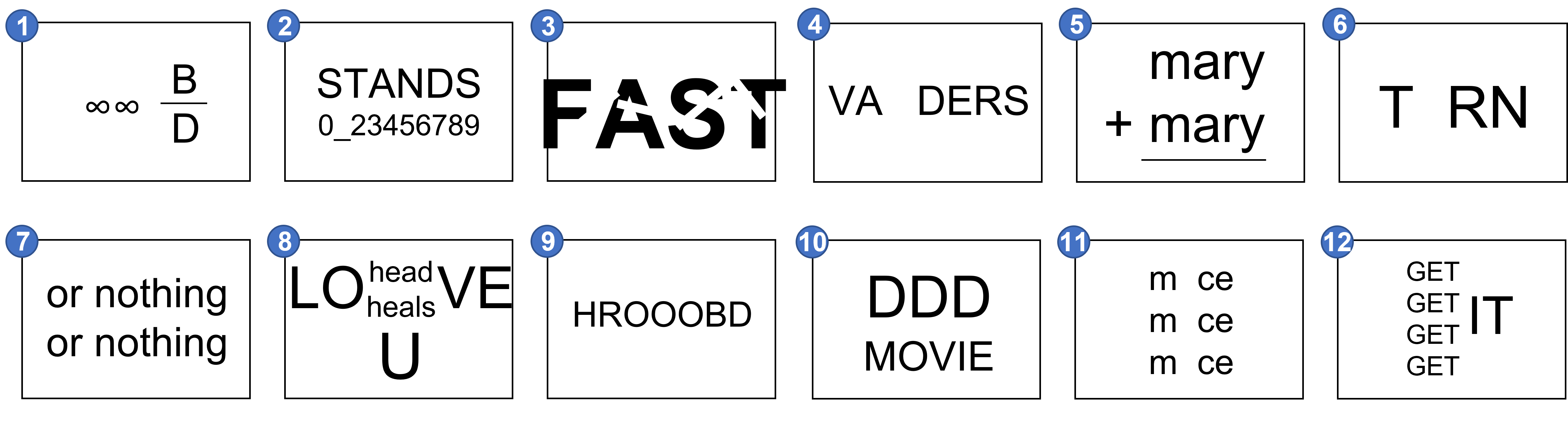1 2 3 4 5 6 7 8 9 10 11 12
Solutions

The solutions to all puzzles will be available on December 25th!

Check back then to verify your solutions! Until then, find somebody else and discuss it with them.

Good Luck!

TBD

TBD

About - 24 Days of Puzzles
24 Days of Puzzles - A new puzzle every day for the first 24 days of December. There will be word puzzles, number puzzles, logic puzzles and more! The puzzles are simply stated and understood, but that doesn't mean they will be easily solved - some may take a bit of thought and time.

For each puzzle there will be a PDF file that can be easily printed and posted.

Most of the puzzles will automatically check your solutions. All solutions will be provided at the end of the month. Until then, check your solutions with someone else to see if they're correct!